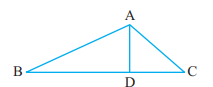### Chapter - 4 Basic Geometrical Ideas

Q
##### Basic Geometrical Ideas

Question:

1. Draw a rough sketch of a triangle ABC. Mark a point P in its interior and a point Q in its exterior. Is the point A in its exterior or in its interior?

Solution:Point A lie on the triangle ABC, neither exterior nor interior of the triangle.

2. (a) Identify three triangles in the figure.

(b) Write the names of seven angles.

(c) Write the names of six line segments.

(d) Which two triangles have ∠B as common?Solution: (a) The three triangles are: ABC, ADC and ADB.

(b) BDA, BCA, BAD, BAC, CBA, CDA and CAB

(c)

(d) ABC and ABD  have ∠B as the common angle.